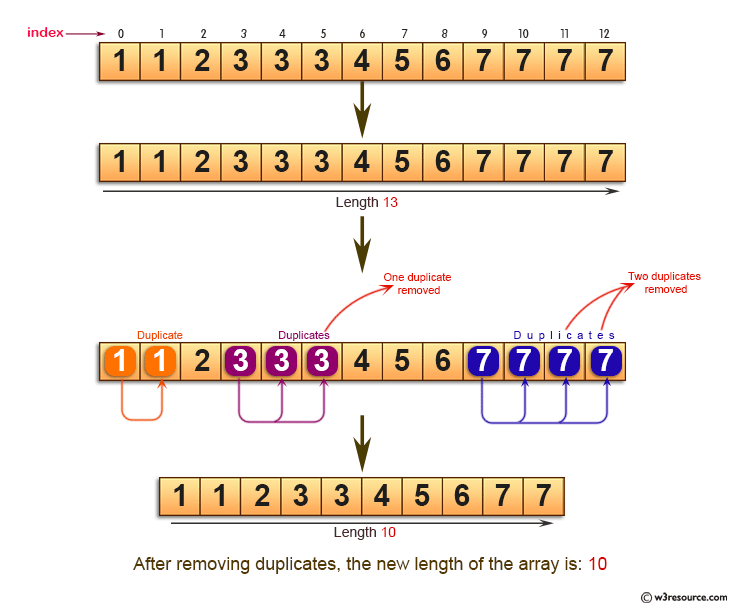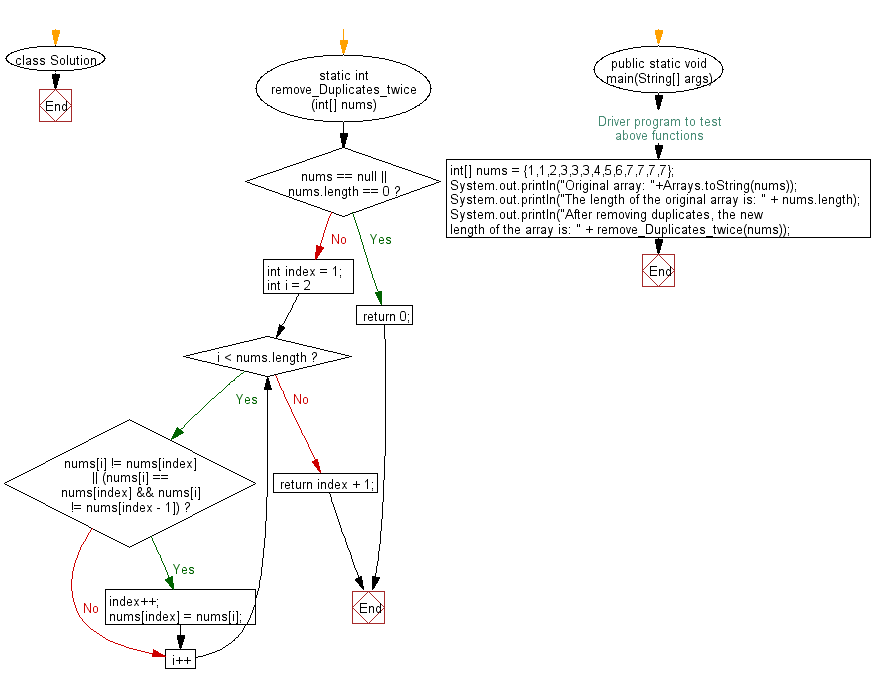﻿ Java exercises: Find the new length of a given sorted array - w3resource# Java Exercises: Find the new length of a given sorted array

## Java Basic: Exercise-132 with Solution

Write a Java program to find the new length of a given sorted array where duplicate elements appeared at most twice.

Pictorial Presentation:Sample Solution:

Java Code:

``````import java.util.Arrays;

class Solution {
static int remove_Duplicates_twice(int[] nums) {
if (nums == null || nums.length == 0) {
return 0;
}

int index = 1;
for (int i = 2; i < nums.length; i++) {
if (nums[i] != nums[index] || (nums[i] == nums[index] && nums[i] != nums[index - 1])) {
index++;
nums[index] = nums[i];
}
}
return index + 1;
}

/* Driver program to test above functions */
public static void main(String[] args)
{
int[] nums = {1,1,2,3,3,3,4,5,6,7,7,7,7};
System.out.println("Original array: "+Arrays.toString(nums));
System.out.println("The length of the original array is: " + nums.length);
System.out.println("After removing duplicates, the new length of the array is: " + remove_Duplicates_twice(nums));
}
}
```
```

Sample Output:

```Original array: [1, 1, 2, 3, 3, 3, 4, 5, 6, 7, 7, 7, 7]
The length of the original array is: 13
After removing duplicates, the new length of the array is: 10
```

Flowchart:Java Code Editor:

What is the difficulty level of this exercise?

Test your Programming skills with w3resource's quiz.

﻿

## Java: Tips of the Day

countOccurrences

Counts the occurrences of a value in an array.

Use Arrays.stream().filter().count() to count total number of values that equals the specified value.

```public static long countOccurrences(int[] numbers, int value) {
return Arrays.stream(numbers)
.filter(number -> number == value)
.count();
}
```

Ref: https://bit.ly/3kCAgLb